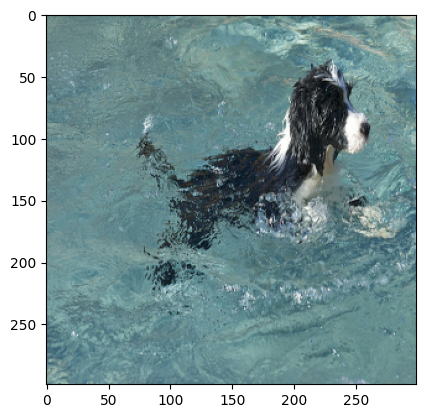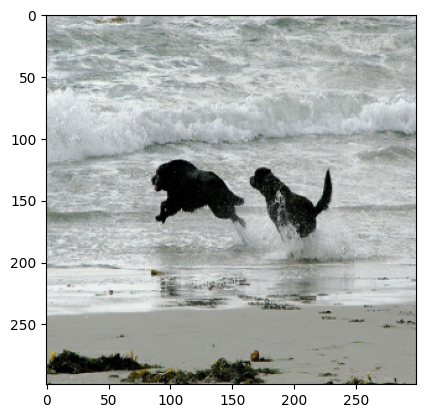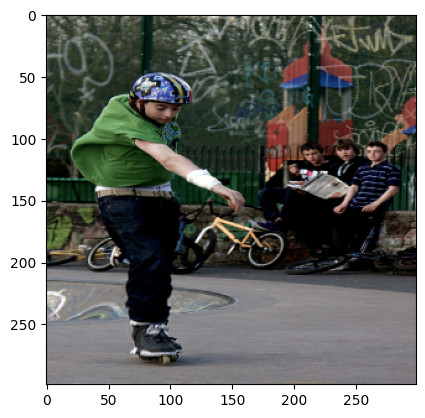» Code examples / Computer Vision / Image Captioning

# Image Captioning

Author: A_K_Nain
Date created: 2021/05/29
Description: Implement an image captioning model using a CNN and a Transformer.View in ColabGitHub source

## Setup

``````import os
import re
import numpy as np
import matplotlib.pyplot as plt

import tensorflow as tf
from tensorflow import keras
from tensorflow.keras import layers
from tensorflow.keras.applications import efficientnet
from tensorflow.keras.layers import TextVectorization

seed = 111
np.random.seed(seed)
tf.random.set_seed(seed)
``````

We will be using the Flickr8K dataset for this tutorial. This dataset comprises over 8,000 images, that are each paired with five different captions.

``````!wget -q https://github.com/jbrownlee/Datasets/releases/download/Flickr8k/Flickr8k_Dataset.zip
!unzip -qq Flickr8k_Dataset.zip
!unzip -qq Flickr8k_text.zip
!rm Flickr8k_Dataset.zip Flickr8k_text.zip
``````
``````# Path to the images
IMAGES_PATH = "Flicker8k_Dataset"

# Desired image dimensions
IMAGE_SIZE = (299, 299)

# Vocabulary size
VOCAB_SIZE = 10000

# Fixed length allowed for any sequence
SEQ_LENGTH = 25

# Dimension for the image embeddings and token embeddings
EMBED_DIM = 512

# Per-layer units in the feed-forward network
FF_DIM = 512

# Other training parameters
BATCH_SIZE = 64
EPOCHS = 30
AUTOTUNE = tf.data.AUTOTUNE
``````

## Preparing the dataset

``````def load_captions_data(filename):
"""Loads captions (text) data and maps them to corresponding images.

Args:
filename: Path to the text file containing caption data.

Returns:
caption_mapping: Dictionary mapping image names and the corresponding captions
text_data: List containing all the available captions
"""

with open(filename) as caption_file:
caption_mapping = {}
text_data = []
images_to_skip = set()

for line in caption_data:
line = line.rstrip("\n")
# Image name and captions are separated using a tab
img_name, caption = line.split("\t")

# Each image is repeated five times for the five different captions.
# Each image name has a suffix `#(caption_number)`
img_name = img_name.split("#")
img_name = os.path.join(IMAGES_PATH, img_name.strip())

# We will remove caption that are either too short to too long
tokens = caption.strip().split()

if len(tokens) < 5 or len(tokens) > SEQ_LENGTH:
continue

if img_name.endswith("jpg") and img_name not in images_to_skip:
# We will add a start and an end token to each caption
caption = "<start> " + caption.strip() + " <end>"
text_data.append(caption)

if img_name in caption_mapping:
caption_mapping[img_name].append(caption)
else:
caption_mapping[img_name] = [caption]

for img_name in images_to_skip:
if img_name in caption_mapping:
del caption_mapping[img_name]

return caption_mapping, text_data

def train_val_split(caption_data, train_size=0.8, shuffle=True):
"""Split the captioning dataset into train and validation sets.

Args:
caption_data (dict): Dictionary containing the mapped caption data
train_size (float): Fraction of all the full dataset to use as training data
shuffle (bool): Whether to shuffle the dataset before splitting

Returns:
Traning and validation datasets as two separated dicts
"""

# 1. Get the list of all image names
all_images = list(caption_data.keys())

# 2. Shuffle if necessary
if shuffle:
np.random.shuffle(all_images)

# 3. Split into training and validation sets
train_size = int(len(caption_data) * train_size)

training_data = {
img_name: caption_data[img_name] for img_name in all_images[:train_size]
}
validation_data = {
img_name: caption_data[img_name] for img_name in all_images[train_size:]
}

# 4. Return the splits
return training_data, validation_data

# Split the dataset into training and validation sets
train_data, valid_data = train_val_split(captions_mapping)
print("Number of training samples: ", len(train_data))
print("Number of validation samples: ", len(valid_data))
``````
``````Number of training samples:  6114
Number of validation samples:  1529
Number of training samples:  6114
Number of validation samples:  1529
``````

## Vectorizing the text data

We'll use the `TextVectorization` layer to vectorize the text data, that is to say, to turn the original strings into integer sequences where each integer represents the index of a word in a vocabulary. We will use a custom string standardization scheme (strip punctuation characters except `<` and `>`) and the default splitting scheme (split on whitespace).

``````def custom_standardization(input_string):
lowercase = tf.strings.lower(input_string)
return tf.strings.regex_replace(lowercase, "[%s]" % re.escape(strip_chars), "")

strip_chars = "!\"#\$%&'()*+,-./:;<=>?@[\]^_`{|}~"
strip_chars = strip_chars.replace("<", "")
strip_chars = strip_chars.replace(">", "")

vectorization = TextVectorization(
max_tokens=VOCAB_SIZE,
output_mode="int",
output_sequence_length=SEQ_LENGTH,
standardize=custom_standardization,
)

# Data augmentation for image data
image_augmentation = keras.Sequential(
[
layers.RandomFlip("horizontal"),
layers.RandomRotation(0.2),
layers.RandomContrast(0.3),
]
)
``````
``````2021-09-17 05:17:57.047819: I tensorflow/stream_executor/cuda/cuda_gpu_executor.cc:937] successful NUMA node read from SysFS had negative value (-1), but there must be at least one NUMA node, so returning NUMA node zero
2021-09-17 05:17:57.058177: I tensorflow/stream_executor/cuda/cuda_gpu_executor.cc:937] successful NUMA node read from SysFS had negative value (-1), but there must be at least one NUMA node, so returning NUMA node zero
2021-09-17 05:17:57.106007: I tensorflow/stream_executor/cuda/cuda_gpu_executor.cc:937] successful NUMA node read from SysFS had negative value (-1), but there must be at least one NUMA node, so returning NUMA node zero
2021-09-17 05:17:57.107650: I tensorflow/core/platform/cpu_feature_guard.cc:142] This TensorFlow binary is optimized with oneAPI Deep Neural Network Library (oneDNN) to use the following CPU instructions in performance-critical operations:  AVX2 FMA
To enable them in other operations, rebuild TensorFlow with the appropriate compiler flags.
2021-09-17 05:17:57.134387: I tensorflow/stream_executor/cuda/cuda_gpu_executor.cc:937] successful NUMA node read from SysFS had negative value (-1), but there must be at least one NUMA node, so returning NUMA node zero
2021-09-17 05:17:57.135154: I tensorflow/stream_executor/cuda/cuda_gpu_executor.cc:937] successful NUMA node read from SysFS had negative value (-1), but there must be at least one NUMA node, so returning NUMA node zero
2021-09-17 05:17:57.135806: I tensorflow/stream_executor/cuda/cuda_gpu_executor.cc:937] successful NUMA node read from SysFS had negative value (-1), but there must be at least one NUMA node, so returning NUMA node zero
2021-09-17 05:17:57.680010: I tensorflow/stream_executor/cuda/cuda_gpu_executor.cc:937] successful NUMA node read from SysFS had negative value (-1), but there must be at least one NUMA node, so returning NUMA node zero
2021-09-17 05:17:57.680785: I tensorflow/stream_executor/cuda/cuda_gpu_executor.cc:937] successful NUMA node read from SysFS had negative value (-1), but there must be at least one NUMA node, so returning NUMA node zero
2021-09-17 05:17:57.681439: I tensorflow/stream_executor/cuda/cuda_gpu_executor.cc:937] successful NUMA node read from SysFS had negative value (-1), but there must be at least one NUMA node, so returning NUMA node zero
2021-09-17 05:17:57.682067: I tensorflow/core/common_runtime/gpu/gpu_device.cc:1510] Created device /job:localhost/replica:0/task:0/device:GPU:0 with 14684 MB memory:  -> device: 0, name: Tesla V100-SXM2-16GB, pci bus id: 0000:00:04.0, compute capability: 7.0
2021-09-17 05:17:58.229404: I tensorflow/compiler/mlir/mlir_graph_optimization_pass.cc:185] None of the MLIR Optimization Passes are enabled (registered 2)
``````

## Building a `tf.data.Dataset` pipeline for training

We will generate pairs of images and corresponding captions using a `tf.data.Dataset` object. The pipeline consists of two steps:

1. Read the image from the disk
2. Tokenize all the five captions corresponding to the image
``````def decode_and_resize(img_path):
img = tf.image.decode_jpeg(img, channels=3)
img = tf.image.resize(img, IMAGE_SIZE)
img = tf.image.convert_image_dtype(img, tf.float32)
return img

def process_input(img_path, captions):
return decode_and_resize(img_path), vectorization(captions)

def make_dataset(images, captions):
dataset = tf.data.Dataset.from_tensor_slices((images, captions))
dataset = dataset.shuffle(BATCH_SIZE * 8)
dataset = dataset.map(process_input, num_parallel_calls=AUTOTUNE)
dataset = dataset.batch(BATCH_SIZE).prefetch(AUTOTUNE)

return dataset

# Pass the list of images and the list of corresponding captions
train_dataset = make_dataset(list(train_data.keys()), list(train_data.values()))

valid_dataset = make_dataset(list(valid_data.keys()), list(valid_data.values()))
``````

## Building the model

Our image captioning architecture consists of three models:

1. A CNN: used to extract the image features
2. A TransformerEncoder: The extracted image features are then passed to a Transformer based encoder that generates a new representation of the inputs
3. A TransformerDecoder: This model takes the encoder output and the text data (sequences) as inputs and tries to learn to generate the caption.
``````def get_cnn_model():
base_model = efficientnet.EfficientNetB0(
input_shape=(*IMAGE_SIZE, 3), include_top=False, weights="imagenet",
)
# We freeze our feature extractor
base_model.trainable = False
base_model_out = base_model.output
base_model_out = layers.Reshape((-1, base_model_out.shape[-1]))(base_model_out)
cnn_model = keras.models.Model(base_model.input, base_model_out)
return cnn_model

class TransformerEncoderBlock(layers.Layer):
def __init__(self, embed_dim, dense_dim, num_heads, **kwargs):
super().__init__(**kwargs)
self.embed_dim = embed_dim
self.dense_dim = dense_dim
)
self.layernorm_1 = layers.LayerNormalization()
self.layernorm_2 = layers.LayerNormalization()
self.dense_1 = layers.Dense(embed_dim, activation="relu")

inputs = self.layernorm_1(inputs)
inputs = self.dense_1(inputs)

attention_output_1 = self.attention_1(
query=inputs,
value=inputs,
key=inputs,
training=training,
)
out_1 = self.layernorm_2(inputs + attention_output_1)
return out_1

class PositionalEmbedding(layers.Layer):
def __init__(self, sequence_length, vocab_size, embed_dim, **kwargs):
super().__init__(**kwargs)
self.token_embeddings = layers.Embedding(
input_dim=vocab_size, output_dim=embed_dim
)
self.position_embeddings = layers.Embedding(
input_dim=sequence_length, output_dim=embed_dim
)
self.sequence_length = sequence_length
self.vocab_size = vocab_size
self.embed_dim = embed_dim
self.embed_scale = tf.math.sqrt(tf.cast(embed_dim, tf.float32))

def call(self, inputs):
length = tf.shape(inputs)[-1]
positions = tf.range(start=0, limit=length, delta=1)
embedded_tokens = self.token_embeddings(inputs)
embedded_tokens = embedded_tokens * self.embed_scale
embedded_positions = self.position_embeddings(positions)
return embedded_tokens + embedded_positions

return tf.math.not_equal(inputs, 0)

class TransformerDecoderBlock(layers.Layer):
def __init__(self, embed_dim, ff_dim, num_heads, **kwargs):
super().__init__(**kwargs)
self.embed_dim = embed_dim
self.ff_dim = ff_dim
)
)
self.ffn_layer_1 = layers.Dense(ff_dim, activation="relu")
self.ffn_layer_2 = layers.Dense(embed_dim)

self.layernorm_1 = layers.LayerNormalization()
self.layernorm_2 = layers.LayerNormalization()
self.layernorm_3 = layers.LayerNormalization()

self.embedding = PositionalEmbedding(
embed_dim=EMBED_DIM, sequence_length=SEQ_LENGTH, vocab_size=VOCAB_SIZE
)
self.out = layers.Dense(VOCAB_SIZE, activation="softmax")

self.dropout_1 = layers.Dropout(0.3)
self.dropout_2 = layers.Dropout(0.5)

def call(self, inputs, encoder_outputs, training, mask=None):
inputs = self.embedding(inputs)

attention_output_1 = self.attention_1(
query=inputs,
value=inputs,
key=inputs,
training=training,
)
out_1 = self.layernorm_1(inputs + attention_output_1)

attention_output_2 = self.attention_2(
query=out_1,
value=encoder_outputs,
key=encoder_outputs,
training=training,
)
out_2 = self.layernorm_2(out_1 + attention_output_2)

ffn_out = self.ffn_layer_1(out_2)
ffn_out = self.dropout_1(ffn_out, training=training)
ffn_out = self.ffn_layer_2(ffn_out)

ffn_out = self.layernorm_3(ffn_out + out_2, training=training)
ffn_out = self.dropout_2(ffn_out, training=training)
preds = self.out(ffn_out)
return preds

input_shape = tf.shape(inputs)
batch_size, sequence_length = input_shape, input_shape
i = tf.range(sequence_length)[:, tf.newaxis]
j = tf.range(sequence_length)
mask = tf.cast(i >= j, dtype="int32")
mult = tf.concat(
[tf.expand_dims(batch_size, -1), tf.constant([1, 1], dtype=tf.int32)],
axis=0,
)

class ImageCaptioningModel(keras.Model):
def __init__(
self, cnn_model, encoder, decoder, num_captions_per_image=5, image_aug=None,
):
super().__init__()
self.cnn_model = cnn_model
self.encoder = encoder
self.decoder = decoder
self.loss_tracker = keras.metrics.Mean(name="loss")
self.acc_tracker = keras.metrics.Mean(name="accuracy")
self.num_captions_per_image = num_captions_per_image
self.image_aug = image_aug

loss = self.loss(y_true, y_pred)

accuracy = tf.equal(y_true, tf.argmax(y_pred, axis=2))
accuracy = tf.cast(accuracy, dtype=tf.float32)

def _compute_caption_loss_and_acc(self, img_embed, batch_seq, training=True):
encoder_out = self.encoder(img_embed, training=training)
batch_seq_inp = batch_seq[:, :-1]
batch_seq_true = batch_seq[:, 1:]
batch_seq_pred = self.decoder(
)
return loss, acc

def train_step(self, batch_data):
batch_img, batch_seq = batch_data
batch_loss = 0
batch_acc = 0

if self.image_aug:
batch_img = self.image_aug(batch_img)

# 1. Get image embeddings
img_embed = self.cnn_model(batch_img)

# 2. Pass each of the five captions one by one to the decoder
# along with the encoder outputs and compute the loss as well as accuracy
# for each caption.
for i in range(self.num_captions_per_image):
loss, acc = self._compute_caption_loss_and_acc(
img_embed, batch_seq[:, i, :], training=True
)

# 3. Update loss and accuracy
batch_loss += loss
batch_acc += acc

# 4. Get the list of all the trainable weights
train_vars = (
self.encoder.trainable_variables + self.decoder.trainable_variables
)

# 6. Update the trainable weights

# 7. Update the trackers
batch_acc /= float(self.num_captions_per_image)
self.loss_tracker.update_state(batch_loss)
self.acc_tracker.update_state(batch_acc)

# 8. Return the loss and accuracy values
return {"loss": self.loss_tracker.result(), "acc": self.acc_tracker.result()}

def test_step(self, batch_data):
batch_img, batch_seq = batch_data
batch_loss = 0
batch_acc = 0

# 1. Get image embeddings
img_embed = self.cnn_model(batch_img)

# 2. Pass each of the five captions one by one to the decoder
# along with the encoder outputs and compute the loss as well as accuracy
# for each caption.
for i in range(self.num_captions_per_image):
loss, acc = self._compute_caption_loss_and_acc(
img_embed, batch_seq[:, i, :], training=False
)

# 3. Update batch loss and batch accuracy
batch_loss += loss
batch_acc += acc

batch_acc /= float(self.num_captions_per_image)

# 4. Update the trackers
self.loss_tracker.update_state(batch_loss)
self.acc_tracker.update_state(batch_acc)

# 5. Return the loss and accuracy values
return {"loss": self.loss_tracker.result(), "acc": self.acc_tracker.result()}

@property
def metrics(self):
# We need to list our metrics here so the `reset_states()` can be
# called automatically.
return [self.loss_tracker, self.acc_tracker]

cnn_model = get_cnn_model()
caption_model = ImageCaptioningModel(
cnn_model=cnn_model, encoder=encoder, decoder=decoder, image_aug=image_augmentation,
)
``````

## Model training

``````# Define the loss function
cross_entropy = keras.losses.SparseCategoricalCrossentropy(
from_logits=False, reduction="none"
)

# EarlyStopping criteria
early_stopping = keras.callbacks.EarlyStopping(patience=3, restore_best_weights=True)

# Learning Rate Scheduler for the optimizer
class LRSchedule(keras.optimizers.schedules.LearningRateSchedule):
def __init__(self, post_warmup_learning_rate, warmup_steps):
super().__init__()
self.post_warmup_learning_rate = post_warmup_learning_rate
self.warmup_steps = warmup_steps

def __call__(self, step):
global_step = tf.cast(step, tf.float32)
warmup_steps = tf.cast(self.warmup_steps, tf.float32)
warmup_progress = global_step / warmup_steps
warmup_learning_rate = self.post_warmup_learning_rate * warmup_progress
return tf.cond(
global_step < warmup_steps,
lambda: warmup_learning_rate,
lambda: self.post_warmup_learning_rate,
)

# Create a learning rate schedule
num_train_steps = len(train_dataset) * EPOCHS
num_warmup_steps = num_train_steps // 15
lr_schedule = LRSchedule(post_warmup_learning_rate=1e-4, warmup_steps=num_warmup_steps)

# Compile the model

# Fit the model
caption_model.fit(
train_dataset,
epochs=EPOCHS,
validation_data=valid_dataset,
callbacks=[early_stopping],
)
``````
``````Epoch 1/30

2021-09-17 05:18:22.943796: I tensorflow/core/kernels/data/shuffle_dataset_op.cc:175] Filling up shuffle buffer (this may take a while): 59 of 256
2021-09-17 05:18:30.137746: I tensorflow/core/kernels/data/shuffle_dataset_op.cc:228] Shuffle buffer filled.
2021-09-17 05:18:30.598020: I tensorflow/stream_executor/cuda/cuda_dnn.cc:369] Loaded cuDNN version 8005

96/96 [==============================] - 62s 327ms/step - loss: 28.1409 - acc: 0.1313 - val_loss: 20.4968 - val_acc: 0.3116
Epoch 2/30

2021-09-17 05:19:13.829127: I tensorflow/core/kernels/data/shuffle_dataset_op.cc:175] Filling up shuffle buffer (this may take a while): 59 of 256
2021-09-17 05:19:19.872802: I tensorflow/core/kernels/data/shuffle_dataset_op.cc:228] Shuffle buffer filled.

96/96 [==============================] - 43s 278ms/step - loss: 19.3393 - acc: 0.3207 - val_loss: 18.0922 - val_acc: 0.3514
Epoch 3/30

2021-09-17 05:19:56.772506: I tensorflow/core/kernels/data/shuffle_dataset_op.cc:175] Filling up shuffle buffer (this may take a while): 61 of 256
2021-09-17 05:20:02.481758: I tensorflow/core/kernels/data/shuffle_dataset_op.cc:228] Shuffle buffer filled.

96/96 [==============================] - 42s 278ms/step - loss: 17.4184 - acc: 0.3552 - val_loss: 17.0022 - val_acc: 0.3698
Epoch 4/30

2021-09-17 05:20:39.367542: I tensorflow/core/kernels/data/shuffle_dataset_op.cc:175] Filling up shuffle buffer (this may take a while): 61 of 256
2021-09-17 05:20:45.149089: I tensorflow/core/kernels/data/shuffle_dataset_op.cc:228] Shuffle buffer filled.

96/96 [==============================] - 43s 278ms/step - loss: 16.3052 - acc: 0.3760 - val_loss: 16.3026 - val_acc: 0.3845
Epoch 5/30

2021-09-17 05:21:21.930582: I tensorflow/core/kernels/data/shuffle_dataset_op.cc:175] Filling up shuffle buffer (this may take a while): 61 of 256
2021-09-17 05:21:27.608503: I tensorflow/core/kernels/data/shuffle_dataset_op.cc:228] Shuffle buffer filled.

96/96 [==============================] - 42s 278ms/step - loss: 15.5097 - acc: 0.3901 - val_loss: 15.8929 - val_acc: 0.3925
Epoch 6/30

2021-09-17 05:22:04.553717: I tensorflow/core/kernels/data/shuffle_dataset_op.cc:175] Filling up shuffle buffer (this may take a while): 61 of 256
2021-09-17 05:22:10.210087: I tensorflow/core/kernels/data/shuffle_dataset_op.cc:228] Shuffle buffer filled.

96/96 [==============================] - 42s 278ms/step - loss: 14.8596 - acc: 0.4069 - val_loss: 15.5456 - val_acc: 0.4005
Epoch 7/30

2021-09-17 05:22:47.100594: I tensorflow/core/kernels/data/shuffle_dataset_op.cc:175] Filling up shuffle buffer (this may take a while): 62 of 256
2021-09-17 05:22:52.466539: I tensorflow/core/kernels/data/shuffle_dataset_op.cc:228] Shuffle buffer filled.

96/96 [==============================] - 42s 277ms/step - loss: 14.3454 - acc: 0.4131 - val_loss: 15.3313 - val_acc: 0.4045
Epoch 8/30

2021-09-17 05:23:29.226300: I tensorflow/core/kernels/data/shuffle_dataset_op.cc:175] Filling up shuffle buffer (this may take a while): 61 of 256
2021-09-17 05:23:34.808841: I tensorflow/core/kernels/data/shuffle_dataset_op.cc:228] Shuffle buffer filled.

96/96 [==============================] - 42s 277ms/step - loss: 13.8745 - acc: 0.4251 - val_loss: 15.2011 - val_acc: 0.4078
Epoch 9/30

2021-09-17 05:24:11.615058: I tensorflow/core/kernels/data/shuffle_dataset_op.cc:175] Filling up shuffle buffer (this may take a while): 62 of 256
2021-09-17 05:24:17.030769: I tensorflow/core/kernels/data/shuffle_dataset_op.cc:228] Shuffle buffer filled.

96/96 [==============================] - 42s 277ms/step - loss: 13.4640 - acc: 0.4350 - val_loss: 15.0905 - val_acc: 0.4107
Epoch 10/30

2021-09-17 05:24:53.832807: I tensorflow/core/kernels/data/shuffle_dataset_op.cc:175] Filling up shuffle buffer (this may take a while): 61 of 256
2021-09-17 05:24:59.506573: I tensorflow/core/kernels/data/shuffle_dataset_op.cc:228] Shuffle buffer filled.

96/96 [==============================] - 42s 277ms/step - loss: 13.0922 - acc: 0.4414 - val_loss: 15.0083 - val_acc: 0.4113
Epoch 11/30

2021-09-17 05:25:36.242501: I tensorflow/core/kernels/data/shuffle_dataset_op.cc:175] Filling up shuffle buffer (this may take a while): 62 of 256
2021-09-17 05:25:41.723206: I tensorflow/core/kernels/data/shuffle_dataset_op.cc:228] Shuffle buffer filled.

96/96 [==============================] - 42s 277ms/step - loss: 12.7538 - acc: 0.4464 - val_loss: 14.9455 - val_acc: 0.4143
Epoch 12/30

2021-09-17 05:26:18.532009: I tensorflow/core/kernels/data/shuffle_dataset_op.cc:175] Filling up shuffle buffer (this may take a while): 62 of 256
2021-09-17 05:26:23.985106: I tensorflow/core/kernels/data/shuffle_dataset_op.cc:228] Shuffle buffer filled.

96/96 [==============================] - 42s 277ms/step - loss: 12.4233 - acc: 0.4547 - val_loss: 14.9816 - val_acc: 0.4133
Epoch 13/30

2021-09-17 05:27:00.696082: I tensorflow/core/kernels/data/shuffle_dataset_op.cc:175] Filling up shuffle buffer (this may take a while): 63 of 256
2021-09-17 05:27:05.812571: I tensorflow/core/kernels/data/shuffle_dataset_op.cc:228] Shuffle buffer filled.

96/96 [==============================] - 42s 277ms/step - loss: 12.1264 - acc: 0.4636 - val_loss: 14.9451 - val_acc: 0.4158
Epoch 14/30

2021-09-17 05:27:42.513445: I tensorflow/core/kernels/data/shuffle_dataset_op.cc:175] Filling up shuffle buffer (this may take a while): 63 of 256
2021-09-17 05:27:47.675342: I tensorflow/core/kernels/data/shuffle_dataset_op.cc:228] Shuffle buffer filled.

96/96 [==============================] - 42s 277ms/step - loss: 11.8244 - acc: 0.4724 - val_loss: 14.9751 - val_acc: 0.4148
Epoch 15/30

2021-09-17 05:28:24.371225: I tensorflow/core/kernels/data/shuffle_dataset_op.cc:175] Filling up shuffle buffer (this may take a while): 63 of 256
2021-09-17 05:28:29.829654: I tensorflow/core/kernels/data/shuffle_dataset_op.cc:228] Shuffle buffer filled.

96/96 [==============================] - 42s 277ms/step - loss: 11.5644 - acc: 0.4776 - val_loss: 15.0377 - val_acc: 0.4167
Epoch 16/30

2021-09-17 05:29:06.564650: I tensorflow/core/kernels/data/shuffle_dataset_op.cc:175] Filling up shuffle buffer (this may take a while): 62 of 256
2021-09-17 05:29:11.945996: I tensorflow/core/kernels/data/shuffle_dataset_op.cc:228] Shuffle buffer filled.

96/96 [==============================] - 42s 277ms/step - loss: 11.3046 - acc: 0.4852 - val_loss: 15.0575 - val_acc: 0.4135

<keras.callbacks.History at 0x7fb3d4b1b1d0>
``````

## Check sample predictions

``````vocab = vectorization.get_vocabulary()
index_lookup = dict(zip(range(len(vocab)), vocab))
max_decoded_sentence_length = SEQ_LENGTH - 1
valid_images = list(valid_data.keys())

def generate_caption():
# Select a random image from the validation dataset
sample_img = np.random.choice(valid_images)

# Read the image from the disk
sample_img = decode_and_resize(sample_img)
img = sample_img.numpy().clip(0, 255).astype(np.uint8)
plt.imshow(img)
plt.show()

# Pass the image to the CNN
img = tf.expand_dims(sample_img, 0)
img = caption_model.cnn_model(img)

# Pass the image features to the Transformer encoder
encoded_img = caption_model.encoder(img, training=False)

# Generate the caption using the Transformer decoder
decoded_caption = "<start> "
for i in range(max_decoded_sentence_length):
tokenized_caption = vectorization([decoded_caption])[:, :-1]
predictions = caption_model.decoder(
)
sampled_token_index = np.argmax(predictions[0, i, :])
sampled_token = index_lookup[sampled_token_index]
if sampled_token == "<end>":
break
decoded_caption += " " + sampled_token

decoded_caption = decoded_caption.replace("<start> ", "")
decoded_caption = decoded_caption.replace(" <end>", "").strip()
print("Predicted Caption: ", decoded_caption)

# Check predictions for a few samples
generate_caption()
generate_caption()
generate_caption()
````````````Predicted Caption:  a group of dogs race in the snow
````````````Predicted Caption:  a man in a blue canoe on a lake
````````````Predicted Caption:  a black and white dog is running through a green grass
``````

## End Notes

We saw that the model starts to generate reasonable captions after a few epochs. To keep this example easily runnable, we have trained it with a few constraints, like a minimal number of attention heads. To improve the predictions, you can try changing these training settings and find a good model for your use case.